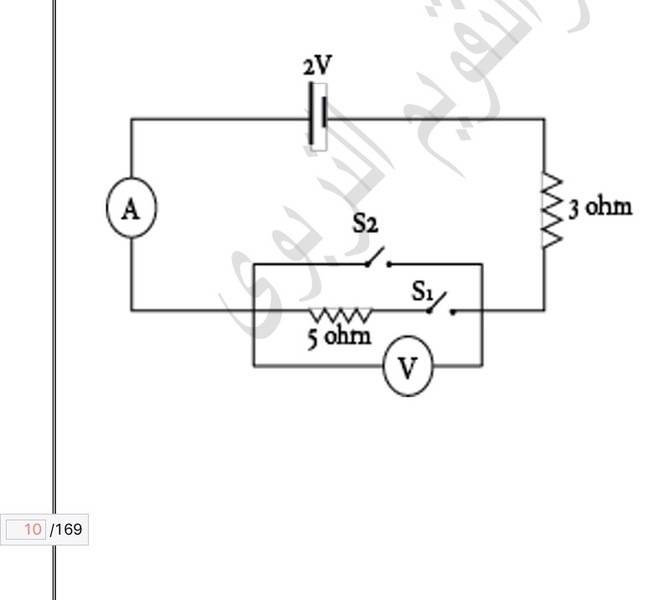# The reading of the ammeter and the reading of the voltmeter.

## Homework Statement

In this circuitWhat will be the reading of the ammeter and that of the voltmeter when:
1. Key S1 and key S2 are open.
2. Key S1 and key S2 are closed.
3. Key S1 is closed and key S2 is open.
(Neglect the internal resistance of the source)

## The Attempt at a Solution

That might be an easy question, but my book doesn't give the solution, and I want to amke sure of my work.

Case 1.
i = 0
V = 2V

Case 2.
i = V/R =2/3 A
V = Vb - iR = 2 - ((2/3) * 3 = 0

Case 3.
Rt = 5+3 = 8 ohm
i = V/Rt = 2/8 = 0.25 A

V = iR = 0.25 * 5 = 1.25 V

Yes, I think you have got all 3 right.

•Ok, but I can't understand why in case two the voltage will be zero? I understand the maths but I don't understand the physics explanation.

Ok, but I can't understand why in case two the voltage will be zero? I understand the maths but I don't understand the physics explanation.
What does S2 do to the 5 ohm resistor?

What does S2 do to the 5 ohm resistor?
S2 cancels the resistance 5 ohm.
But we do still have the resistance 3 ohm.

S2 cancels the resistance 5 ohm.
In technical terms, S2 short-circuits the 5 ohm resistor. So the 5 ohm resistor is ineffective.

In technical terms, S2 short-circuits the 5 ohm resistor. So the 5 ohm resistor is ineffective.
OK, and then what? more explanation please!

OK, and then what? more explanation please!
What is the resistance of S2 and the wire that shorts the 5 ohm resistance?

It is zero so the voltage is zero.
Ok, I got it. But what's your opinion about the whole solution?

It is zero so the voltage is zero.
•